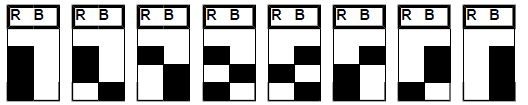# THE FIGURES

What are the figures? For the purpose of the simple combinations of the roulette, a number anyone can be PAR or ODD, LACK or PASSES, RED or BLACK. We will center in this last combination.

We have two columns to note the combination of each play. If the number is red we make an annotation in the cabin of the left and if it is black in the right cabin. Each play can have an alone annotation, or to the right or to the left, and it is one of the two possible alternatives: RED or BLACK. It is said that the possible figures of 1 (of one play) are 2.

In the second consecutive play the possibilities are: RED - RED, RED -BLACK, BLACK - RED, BLACK - BLACK, and is said that the figures of 2 are 4. In 3 consecutive plays the possibilities are 8 (fig. 1).

GRAPHIC 1The possibility of the fact that is formed a figure of 3 (1 between 8) is the half of be formed a figure of 2 (1 between 4) and the fourth part that a figure of 1 (1 between 2). Is the same as to say:

So that could be formed one figure of 2 those of 1 will have been able to form 2 times each one, and so that could be formed one figure of 3 those of 2 will have been able to form also 2 times, and thus successive. For so much, we can say that the figures of 1 are the double that those of 2, the figures of 2 are the double that those of 3, the figures of 3 are the double that those of 4, the figures of 4 are the double that those of 5, etc.

This reasoning can be applied to the series saying that the series of 1 are the double that those of 2, the series of 2 are the double that those of 3, the series of 3 are the double that those of 4, etc.

Evidently this ideal distribution is not fulfilled, and we can only assert is that while goes increasing the number of plays, the distribution will be gone approximating every time more to this ideal distribution. It can be that never it is fulfilled exactly, but the difference between the percentage that would correspond to every series taking into account the number of plays and the real will be every time smaller.

The ideal distribution of the series in 247 plays would be this:

SERIES PLAY %

• 1 series of 7 = 7 plays 0.78
• 2 series of 6 = 12 plays 1.57
• 4 series of 5. = 20 “ 3.15
• 8 series of 4. = 32 “ 6.30
• 16 series of 3. = 48 “ 12.59
• 32 series of 2. = 64 “ 25.19
• 64 series of 1. = 64 “ 50.39

TOTAL 127 series. 247 plays. 100.00

If we go increasing the number of plays the corresponding percentage to the series of 1 goes approximating to 50%, that of the series of 2 to 25%, that of the series of 3 to 12,5%, etc.

## Play the Best LIVE Dealer Casinos### Lab Casino

100% up to €100### Casino Planet

100% up to €300 + 200 FS### 22BET Casino